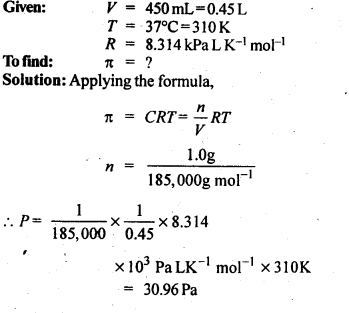# Solutions TEXTBOOK Solution

2.1. Calculate the mass percentage of benzene (C6H6) and carbon tetrachloride (CCl4) if 22 g of benzene is dissolved in 122 g of carbon tetrachloride.

Mass of solution = Mass of C6H6 + Mass of CCl4
= 22 g+122 g= 144 g
Mass % of benzene = 22/144 x 100 =15.28 %
Mass % of CCl4 = 122/144 x 100 = 84.72 %

2.2. Calculate the mole fraction of benzene in solution containing 30% by mass in carbon tetrachloride.

30% by mass of C6H6 in CCl4 => 30 g C6H6 in 100 g solution
.’. no. of moles of C6H6,(nC6h6) = 30/78 = 0.385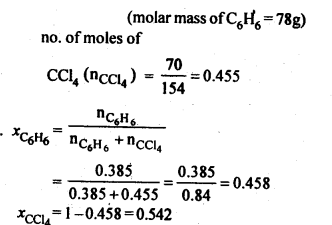2.3. Calculate the molarity of each of the following solutions

(a) 30 g of Co(NO3)26H2O in 4·3 L of solution
(b) 30 mL of 0-5 M H2SO4 diluted to 500 mL.2.4. Calculate the mass of urea (NH2CONH2) required in making 2.5 kg of 0.25 molal aqueous solution.

0.25 Molal aqueous solution to urea means that
moles of urea = 0.25 mole
mass of solvent (NH2CONH2) = 60 g mol-1
.’. 0.25 mole of urea = 0.25 x 60=15g
Mass of solution = 1000+15 = 1015g = 1.015 kg
1.015 kg of urea solution contains 15g of urea
.’. 2.5 kg of solution contains urea =15/1.015 x 2.5 = 37 g

2.5. Calculate

(a) molality
(b) molarity and
(c) mole fraction of KI if the density of 20% (mass/mass) aqueous KI solution is 1·202 g mL-1.

Step I. Calculation of molality of solution

Weight of KI in 100 g of the solution = 20 g
Weight of water in the solution = 100 – 20 = 80 g = 0-08 kg
Molar mass of KI = 39 + 127 = 166 g mol-1.Step II. Calculation of molarity of solution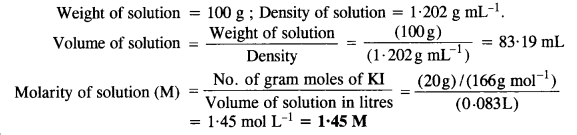Step III. Calculation of mole fraction of Kl2.6. HS, a toxic gas with rotten egg like smell, is used for the qualitative analysis. If the solubility of H2S in water at STP is 0.195 m, calculate Henry’s law constant.

Solubility of H2S gas = 0.195 m
= 0.195 mole in 1 kg of solvent
1 kg of solvent = 1000g2.7. Henry’s law constant for CO2 in water is 1.67 x 10Pa at 298 K. Calculate the quantity of CO2 in 500 mL of soda water when packed under 2.5 atm CO2 pressure at 298 K.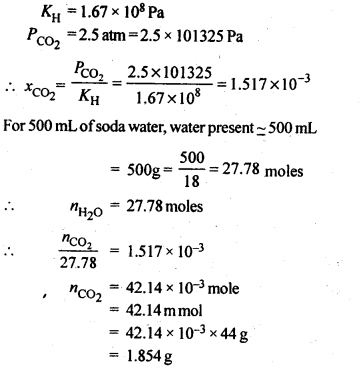2.8 The vapour pressures of pure liquids A and B are 450 mm and 700 mm of Hg respectively at 350 K. Calculate the composition of the liquid mixture if total vapour pressure is 600 mm of Hg. Also find the composition in the vapour phase.

Vapour pressure of pure liquid A (\({ P }_{ A }^{ \circ }\)) = 450 mm
Vapour pressure of pure liquid B (\({ P }_{ B }^{ \circ }\)) = 700 mm
Total vapour pressure of the solution (P) = 600 mm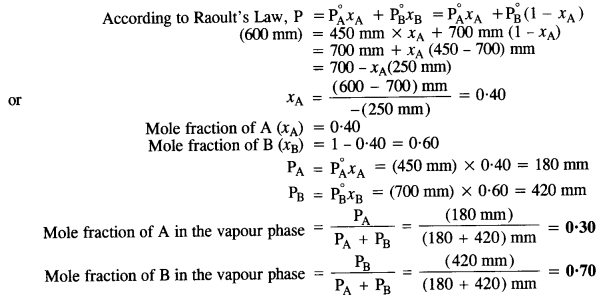2.9. Vapour pressure of pure water at 298 K is 23.8 m m Hg. 50 g of urea (NH2CONH2) is dissolved in 850 g of water. Calculate the vapour pressure of water for this solution and its relative lowering.2.10. Boiling point of water at 750 mm Hg is 99.63°C. How much sucrose is to be added to 500 g of water such that it boils at 100°C.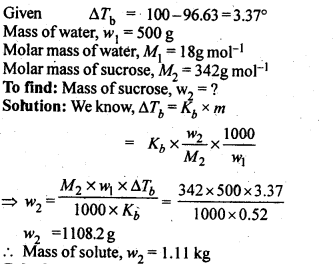2.11 Calculate the mass of ascorbic acid (vitamin C, C6H8O6) to be dissolved in 75 g of acetic acid to lower its melting point by 1·5°C. (Kf for CH3COOH) = 3·9 K kg mol-1)2.12. Calculate the osmotic pressure in pascals exerted by a solution prepared by dissolving 1.0 g of polymer of molar mass 185,000 in 450 mL of water at 37°C.